The KRIGE2D Procedure

Computational Resources

To generate a predicted value at a single grid point by using N data points, PROC KRIGE2D must solve the kriging system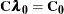where the dimensions of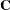are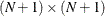and the right-hand-side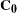has one column.

Holding the matrix and vector associated with this system in core requires approximately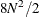bytes. The CPU time used in solving the system is proportional to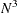. For large N, this time dominates the O(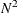) time to compute the elements of the covariance matrix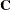from the specified covariance or semivariogram model.

For local kriging, the kriging system is set up and solved for each grid point. Part of the setup process involves determining the neighborhood of each grid point. A fast K-D tree algorithm determines neighborhoods. For G grid points, the dominant CPU time factor is setting up and solving the G kriging systems. The N in the algorithm of the section Ordinary Kriging is the number of data points in a given neighborhood, and it can differ for each grid point.

In global kriging, the entire input data set and all grid points set up and solve the single systemAgainhas dimensions, but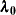andnow have G columns, where G is the number of grid points. Memory requirements are approximately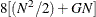bytes. The CPU time used in solving the system is still dominated by thefactorization of the left-hand side.